Updating search results...

# 18 Results

View
Selected filters:
• CCSS.Math.Content.7.SP.C.6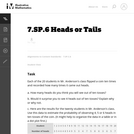Unrestricted Use
CC BY
Rating
0.0 stars

This is a task from the Illustrative Mathematics website that is one part of a complete illustration of the standard to which it is aligned. Each task has at least one solution and some commentary that addresses important asects of the task and its potential use. Here are the first few lines of the commentary for this task: Each of the 20 students in Mr. Anderson's class flipped a coin ten times and recorded how many times it came out heads. How many heads do you think you...

Subject:
Mathematics
Material Type:
Activity/Lab
Provider:
Illustrative Mathematics
Provider Set:
Illustrative Mathematics
Author:
Illustrative Mathematics
05/22/2013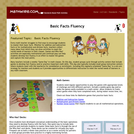Rating
0.0 stars

Every math teacher struggles to find ways to encourage students to master their basic facts. Whether for addition and subtraction facts or for multiplication and division facts, teachers collect many ideas from which they can draw activities to meet the varied needs of learners in their classes. Games and Who Has? activities are especially motivational and continual play can help students develop fact fluency in an effort to master the games and capture the most points.

Material Type:
Activity/Lab
Game
Provider:
Mathwire
Author:
Terry Kawas
02/16/2011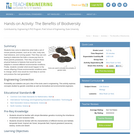Educational Use
Rating
0.0 stars

Students toss coins to determine what traits a set of mouse parents possess, such as fur color, body size, heat tolerance, and running speed. Then they use coin tossing to determine the traits a mouse pup born to these parents possesses. Then they compare these physical features to features that would be most adaptive in several different environmental conditions. Finally, students consider what would happen to the mouse offspring if those environmental conditions were to change: which mice would be most likely to survive and produce the next generation?

Subject:
Applied Science
Engineering
Genetics
Life Science
Material Type:
Activity/Lab
Provider:
TeachEngineering
Provider Set:
TeachEngineering
Author:
Mary R. Hebrank
10/14/2015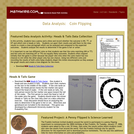Rating
0.0 stars

Using two different coins and recording the results of both coins helps students dispel this initial misconception as they analyze the graph results. Class discussion should focus on analyzing the data to determine if the game is fair or not. Directions and gameboard are included in the download.

Material Type:
Game
Provider:
Mathwire
Author:
Terry Kawas
02/16/2011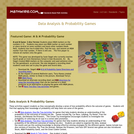Rating
0.0 stars

These activities support students as they conceptually develop a sense of how probability affects the outcome of games. Students will find that applying their knowledge of probability will help them win some of the games

Material Type:
Game
Provider:
Mathwire
Author:
Terry Kawas
02/16/2011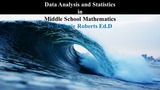Unrestricted Use
CC BY
Rating
0.0 stars

This supplemental resource provides problems and activities related to Data Analysis and Statistics in Middle School Mathematics.

Subject:
Mathematics
Material Type:
Activity/Lab
Author:
Twianie Roberts Ed.D
09/25/2022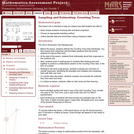Only Sharing Permitted
CC BY-NC-ND
Rating
0.0 stars

This lesson unit is intended to help you assess how well students are able to: solve simple problems involving ratio and direct proportion; choose an appropriate sampling method; and collect discrete data and record them using a frequency table.

Subject:
Education
Geometry
Mathematics
Measurement and Data
Ratios and Proportions
Material Type:
Assessment
Lecture Notes
Lesson Plan
Teaching/Learning Strategy
Provider:
Shell Center for Mathematical Education
Provider Set:
Mathematics Assessment Project (MAP)
Author:
http://map.mathshell.org/
04/26/2013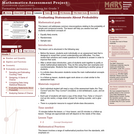Only Sharing Permitted
CC BY-NC-ND
Rating
0.0 stars

This lesson unit addresses common misconceptions relating to probability of simple and compound events. The lesson will help you assess how well students understand concepts of: Equally likely events; randomness; and sample sizes.

Subject:
Mathematics
Statistics and Probability
Material Type:
Assessment
Lesson Plan
Provider:
Shell Center for Mathematical Education
Provider Set:
Mathematics Assessment Project (MAP)
04/26/2013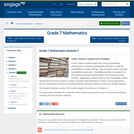Conditional Remix & Share Permitted
CC BY-NC-SA
Rating
0.0 stars

In this module, students begin their study of probability, learning how to interpret probabilities and how to compute probabilities in simple settings.  They also learn how to estimate probabilities empirically.  Probability provides a foundation for the inferential reasoning developed in the second half of this module.  Additionally, students build on their knowledge of data distributions that they studied in Grade 6, compare data distributions of two or more populations, and are introduced to the idea of drawing informal inferences based on data from random samples.

Subject:
Mathematics
Statistics and Probability
Material Type:
Module
Provider:
New York State Education Department
Provider Set:
EngageNY
01/06/2014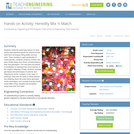Educational Use
Rating
0.0 stars

Students randomly select jelly beans (or other candy) that represent genes for several human traits such as tongue-rolling ability and eye color. Then, working in pairs (preferably of mixed gender), students randomly choose new pairs of jelly beans from those corresponding to their own genotypes. The new pairs are placed on toothpicks to represent the chromosomes of the couple's offspring. Finally, students compare genotypes and phenotypes of parents and offspring for all the "couples" in the class. In particular, they look to see if there are cases where parents and offspring share the exact same genotype and/or phenotype, and consider how the results would differ if they repeated the simulation using more than four traits.

Subject:
Applied Science
Engineering
Genetics
Life Science
Material Type:
Activity/Lab
Lesson Plan
Provider:
TeachEngineering
Provider Set:
TeachEngineering
Author:
Mary R. Hebrank
09/26/2008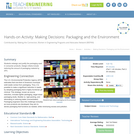Educational Use
Rating
0.0 stars

Students redesign and justify the packaging used in consumer products. Design criteria include reducing the amount of packaging material by 25%.

Subject:
Applied Science
Engineering
Environmental Science
Material Type:
Activity/Lab
Provider:
TeachEngineering
Provider Set:
TeachEngineering
Author:
K. M. Samuelson
Martha Cyr
09/18/2014Conditional Remix & Share Permitted
CC BY-NC
Rating
0.0 stars

Four full-year digital course, built from the ground up and fully-aligned to the Common Core State Standards, for 7th grade Mathematics. Created using research-based approaches to teaching and learning, the Open Access Common Core Course for Mathematics&nbsp;is designed with student-centered learning in mind, including activities for students to develop valuable 21st century skills and academic mindset.

Subject:
Mathematics
Material Type:
Full Course
Provider:
Pearson
10/06/2016Conditional Remix & Share Permitted
CC BY-NC
Rating
0.0 stars

Students choose a project idea and a partner or group. They write a proposal for their project.Key ConceptsProjects engage students in the applications of mathematics. It is important for students to apply mathematical ways of thinking to solve rich problems. Students are more motivated to understand mathematical concepts if they are engaged in solving a problem of their own choosing. In this lesson, students are challenged to identify an interesting mathematical problem and to choose a partner or a group to work collaboratively on solving that problem. Students gain valuable skills in problem solving, reasoning, and communicating mathematical ideas with others.Goals and Learning ObjectivesIdentify a project idea.Identify a partner or group to work collaboratively on a math project.

Subject:
Mathematics
Material Type:
Lesson Plan
09/21/2015Conditional Remix & Share Permitted
CC BY-NC
Rating
0.0 stars

Samples and ProbabilityType of Unit: ConceptualPrior KnowledgeStudents should be able to:Understand the concept of a ratio.Write ratios as percents.Describe data using measures of center.Display and interpret data in dot plots, histograms, and box plots.Lesson FlowStudents begin to think about probability by considering the relative likelihood of familiar events on the continuum between impossible and certain. Students begin to formalize this understanding of probability. They are introduced to the concept of probability as a measure of likelihood, and how to calculate probability of equally likely events using a ratio. The terms (impossible, certain, etc.) are given numerical values. Next, students compare expected results to actual results by calculating the probability of an event and conducting an experiment. Students explore the probability of outcomes that are not equally likely. They collect data to estimate the experimental probabilities. They use ratio and proportion to predict results for a large number of trials. Students learn about compound events. They use tree diagrams, tables, and systematic lists as tools to find the sample space. They determine the theoretical probability of first independent, and then dependent events. In Lesson 10 students identify a question to investigate for a unit project and submit a proposal. They then complete a Self Check. In Lesson 11, students review the results of the Self Check, solve a related problem, and take a Quiz.Students are introduced to the concept of sampling as a method of determining characteristics of a population. They consider how a sample can be random or biased, and think about methods for randomly sampling a population to ensure that it is representative. In Lesson 13, students collect and analyze data for their unit project. Students begin to apply their knowledge of statistics learned in sixth grade. They determine the typical class score from a sample of the population, and reason about the representativeness of the sample. Then, students begin to develop intuition about appropriate sample size by conducting an experiment. They compare different sample sizes, and decide whether increasing the sample size improves the results. In Lesson 16 and Lesson 17, students compare two data sets using any tools they wish. Students will be reminded of Mean Average Deviation (MAD), which will be a useful tool in this situation. Students complete another Self Check, review the results of their Self Check, and solve additional problems. The unit ends with three days for students to work on Gallery problems, possibly using one of the days to complete their project or get help on their project if needed, two days for students to present their unit projects to the class, and one day for the End of Unit Assessment.

Subject:
Mathematics
Statistics and Probability
Material Type:
Unit of Study
Provider:
PearsonConditional Remix & Share Permitted
CC BY-NC
Rating
0.0 stars

Students begin to formalize their understanding of probability. They are introduced to the concept of probability as a measure of likelihood and how to calculate probability as a ratio. The terms discussed (impossible, certain, etc.) in Lesson 1 are given numerical values.Key ConceptsStudents will think of probability as a ratio; it can be written as a fraction, decimal, or a percent ranging from 0 to 1.Students will think about ratio and proportion to predict results.Goals and Learning ObjectivesDefine probability as a measure of likelihood and the ratio of favorable outcomes to the total number of outcomes for an event.Predict results based on theoretical probability using ratio and proportion.

Subject:
Statistics and Probability
Material Type:
Lesson Plan
09/21/2015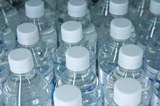Conditional Remix & Share Permitted
CC BY-NC
Rating
0.0 stars

Students will extend their understanding of probability by continuing to conduct experiments with outcomes that do not have a theoretical probability. They will make predictions on the number of outcomes from a series of trials, and compare their predictions with the experimental probability calculated from an experiment.Key ConceptsStudents continue to investigate the Law of Large Numbers.Goals and Learning ObjectivesDeepen understanding of experimental probability.Use proportions to predict results for a number of trials and to calculate experimental probability.Understand that some events do not have theoretical probability.Understand that there are often many factors involved in determining probability (e.g., human error, randomness).

Subject:
Statistics and Probability
Material Type:
Lesson Plan
09/21/2015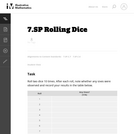Unrestricted Use
CC BY
Rating
0.0 stars

his task is intended as a classroom activity. Student pool the results of many repetitions of the random phenomenon (rolling dice) and compare their results to the theoretical expectation they develop by considering all possible outcomes of rolling two dice. This gives them a concrete example of what we mean by long term relative frequency.

Subject:
Mathematics
Statistics and Probability
Material Type:
Activity/Lab
Provider:
Illustrative Mathematics
Provider Set:
Illustrative Mathematics
Author:
Illustrative Mathematics
10/09/2012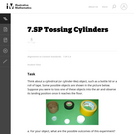Unrestricted Use
CC BY
Rating
0.0 stars

The purpose of this task is to provide students with the opportunity to determine experimental probabilities by collecting data.

Subject:
Mathematics
Statistics and Probability
Material Type:
Activity/Lab
Provider:
Illustrative Mathematics
Provider Set:
Illustrative Mathematics
Author:
Illustrative Mathematics